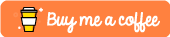This post is going to explain what a computed property is and how to use it.

There is a Swift Playground with examples in this post. It can be found at this link. Feel free to copy the files or clone the entire repo, but the repo has more than just this post's sample code.

## Topics covered in this post

• What is a Computed Property
• Examples using getters and Setters
• Why use a Computed Property

# What is a Computed Property?

A computed property is similar to a stored property, except a computed property does not hold a value. A computed property's value is calculated based on the provided getter defined for the property when the computed property is called.

A computed property can also affect other properties in the class, struct, or enumeration by using a setter.

## Important Things about Computed Properties

• Always declare computed properties as a variable, `var` . A computed property is never declared as a constant, `let` , since the values are never fixed.
• The code inside the getter runs every time the property is called.
• Setters can affect other properties
• When declaring a computed property, a type has to be specified

## Example of a computed property with only a getter

``````struct Example {
// 1.
var originalNum: Int = 1
// 2.
// 3.
get {
return originalNum + 2
}
}
}
``````

Here's an explanation of what happens in the example above.

`orginalNum` is a stored property that will affect `addTwo`

`addTwo` is the computed property. Every time `addTwo` is called it will run the code inside the getter. Notice that `addTwo` has a type of `Int` .

`get` is optional if the computed property is read-only. `addTwo` could have been written as:

``````var addTwo: Int {
retun orginalNum + 2
}
``````

## Another example

``````struct Rectangle {
var width: Int
var height: Int

var area: Int{
get {
return width*height
}
}
}

var rect: Rectangle = Rectangle(width: 12, height: 5)
rect.area // 60
``````

In the example above, `area` is a computed property. If `rect.width` , changes to 15, then `area` will change the next time it's called, which is seen in the code below.

``````rect.width = 15
rect.area // 75
``````

## Example of the computed property with a getter and setter

``````struct Square {
var width: Int
// 1.
var area: Int {
get {
return width * width
}
// 2.
set(newArea) {
// 3.
width = Int(sqrt(Double(newArea)))
}
}
}

var sqr: Square = Square(width: 12)
sqr.area // 144
sqr.area = 25
sqr.width // 5
``````

Here's an explanation of what happens in the example above.

1. Declares the computed property as a variable as an Int

2. Declares the setter that will take an Int called `newArea`

3. Inside the setter, the width is assigned the square root of the `newArea`

# Why use a Computed Property

A computed property is useful when wanting to recall a value that can be calculated from other properties in the class, structure, or enumeration.

In the `Square` structure, `area` is a computed property because the area is always dependent on the width of the square. `area` could have also been written as two functions, one to set the width based on the `newArea` and another to get the area. Since, both would effect the width and gets/sets a value, a computed property makes a bit more sense and makes the code look a bit cleaner.

When choosing between a computed property and a function, keep in mind the cost of the operation. Every time a computed property is called, it is recalculated. Also, a computed property can't take any parameters when getting a value. The example below shows a computed property and a function.

``````struct Circle {
// 1.
var area: Double {
get {
}
set(newArea) {
}
}
// 2.
func getBiggerCirclesArea(times: Int) -> Double{
return radius * Double(times) * Double.pi
}
}
``````

Here's an explanation for the code above.

`area` is a computed property. It looks similar to `Square` 's area, but it has a circle formula. A computed property works for `area` since the radius of the circle might change,

`getBiggerCirclesArea` is a function. Having it be a function will allow the bigger circle be specified without adjusting the original `Circle` 's radius.

If you enjoyed this post and want more, please consider subscribing to my Patreon and checking out my other posts!

If you don't like having more subscriptions, consider buying me a coffee by clicking the image below!This post is also available on DEV.